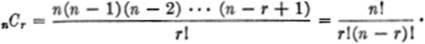﻿ ﻿Reference Material from Other Branches of Mathematics - The Calculus Primer

## The Calculus Primer (2011)

### Reference Material from Other Branches of Mathematics

ALGEBRA

FACTORS:

a2 ± 2ab + b2 = (a ± 6)2

a2b2 = (a + b)(a − b)

a3 ± b3 = (a ± b)(a2 / ab + b2)

a4b4 = (a2 + b2) (a + b) (ab)

a4 + b4 = (a2 + b2 +ab)(a2 + b2ab)

a2n − b2n = (an + bn)(anbn)

EXPONENTS:ROOTS:LOGARITHMS:If r1 and r2 are the roots of the equation

ax2 + bx + c = 0,The discriminant Δ = b2 − 4ac;

if Δ > 0, the roots are real and distinct;

if Δ = 0, the roots are real and equal;

if Δ < 0, the roots are complex.

(xr1) (xr2) = ax2 + bx + c = 0;PROGRESSIONS:

Arithmetic.l = a + (n − 1) d;Geometric.l = arn−1;COMPLEX NUMBERS:Ifa + bi = x + yi,then a = x and b = y.

Ifa + bi = r(cos θ + i sin θ),

thena = r cos θ,b = r sin θ,[r(cos θ + i sin θ)]n = rn(cos nθ + i sin nθ).

FACTORIALS:

n! = 1·2·3·4 ··· to n factors.

nPn = n!0! = 1nCr = nCn−r

nCn = nC0 = 1

nC1 + nC2 + nC3 + ··· + nCn = 2n − 1;

nC0 + nC1 + nC2 + ··· + nCn = 2n.

BINOMIAL EXPANSION:The rth term of (a + b)n is:DETERMINANTS:ZERO AND INFINITY:

The symbols 0 and ∞ are not to be regarded as “numbers” in these formulas:GEOMETRY

In the following formulas,

b = length of base

h = altitude

l = slant height

m = median

d = diameter

P = perimeter

C = circumference

S = arc length

s = semiperimeter

K = area

B = area of base

V = volumeTriangle: P = a + b + c

K =bh =Trapezoid: m =(b1 + b2)

K =h(b1 + b2) =mh

Circle: C = πd = 2πrSector: S =

K =r2θ

Prism: V = Bh

Cylinder: (right circular) K = 2πrh + 2πr2

V = Bh = πr2h

Pyramid: V =Bh

Cone: (right circular) K = πrl + πr2; l =V =Bh =πr2h

Sphere: K = 4πr2

V =πr3

Spherical triangle: K =, where E = (a° + b° + c°) − 180°.

TRIGONOMETRY

FUNDAMENTAL IDENTITIES:sin2x + cos2 x = 1;sec2 x = 1 + tan2 x;csc2 x = 1 + cot2 x.

TRANSFORMATIONS:

Functions of the Sum and Difference of Two Angles:

sin (x ± y) = sin x cos y ± cos x sin y,

cos (x ± y) = cos x cos y / sin x sin y,Multiple Angle Formulas:

sin 2x = 2 sin x cos x,

cos 2x = cos2 x − sin2 x = 2 cos2 x − 1 = 1 − 2 sin2 x,Sum and Product Formulas:

sin x + sin y = 2 sin(x + y) cos(xy),
sin x − sin y = 2 cos(x + y) sin(xy),
cos x + cos y = 2 cos(x + y) cos (xy),
cos x − cos y = −2 sin(x + y) sin(xy),
sin x sin y =cos (xy) −cos (x + y),
sin x cos y =sin (xy) +sin (x + y),
cos x cos y =cos (xy) + cos (x + y).

sin2 x − sin2 y = sin (x + y) sin (xy),
cos2 x − cos2 y = − sin (x + y) sin (xy),
cos2 x − sin2 y = cos (x + y) cos (xy),

sin2 x =cos 2x,
cos2 x =+cos 2x.

PLANE TRIANGLES:

a, b, c = sides of the triangle

A, B, C = opposite angles of the triangleR = radius of the circumscribed circle

K = area of the triangleFUNCTIONS OF SPECIAL ANGLES:PLANE ANALYTIC GEOMETRY

FUNDAMENTAL RELATIONS:EQUATIONS OF A STRAIGHT LINE:POLAR COORDINATES:

If P(x,y) ≡ P(r,θ), thenCONIC SECTIONS:CURVES FOR REFERENCESOLID ANALYTIC GEOMETRY

FUNDAMENTAL RELATIONSEQUATIONS OF A PLANE:EQUATIONS OF A LINE:(1)Sphere with center at (0,0,0):

x2 + y2 + z2 = r2.

(2)Ellipsoid with center at (0,0,0):(3)Hyperboloid of one sheet:(4)Hyperboloid of two sheets:(5)Elliptic Paraboloid:GREEK ALPHABET﻿